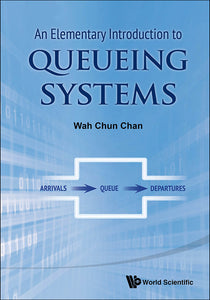# Elementary Introduction To Queueing Systems, An

Wah Chun Chan

Format: Print Book

ISBN: 9789814612005

• SGD 94.16
Unit price per
Tax included.

The book aims to highlight the fundamental concepts of queueing systems. It starts with the mathematical modeling of the arrival process (input) of customers to the system. It is shown that the arrival process can be described mathematically either by the number of arrival customers in a fixed time interval, or by the interarrival time between two consecutive arrivals. In the analysis of queueing systems, the book emphasizes the importance of exponential service time of customers. With this assumption of exponential service time, the analysis can be simplified by using the birth and death process as a model. Many queueing systems can then be analyzed by choosing the proper arrival rate and service rate. This facilitates the analysis of many queueing systems.Drawing on the author's 30 years of experience in teaching and research, the book uses a simple yet effective model of thinking to illustrate the fundamental principles and rationale behind complex mathematical concepts. Explanations of key concepts are provided, while avoiding unnecessary details or extensive mathematical formulas. As a result, the text is easy to read and understand for students wishing to master the core principles of queueing theory.

Format: Hardcover
No of Pages: 116
Imprint: World Scientific
Publication date: 20140530